# Circles to Circles

This post expands on something I said in passing yesterday. I said in the body of the post that

… the image of a circle in the complex plane under a Möbius transformation is another circle.

and added in a footnote that

For this to always be true, you have to include a line as a special case of a circle, a circle of infinite radius if you like.

This post will illustrate these statements with Python code and plots. First, some code for drawing circles and other curves in the complex plane.

```    from numpy import exp, pi, linspace
import matplotlib.pyplot as plt

θ = linspace(0, 2*pi, 200)

def plot_curves(curves):
for c in curves:
plt.plot(c.real, c.imag)
plt.axes().set_aspect(1)
plt.show()
plt.close()
```

Next, code for Möbius transformations, and the particular Möbius transformation we’ll use in our plots.

```    def mobius(z, a, b, c, d):
return (a*z + b)/(c*z + d)

def m(curve):
return mobius(curve, 1, 2, 3, 4)
```

Now we’ll plot three circles and their images under the Möbius transformation

m(z) = (z + 2)/(3z + 4)

with the following code.

```    circles = [circle(1, 0), circle(2, 0), circle(2, 2)]
plot_curves(circles)
plot_curves([m(c) for c in circles])
```

This producesand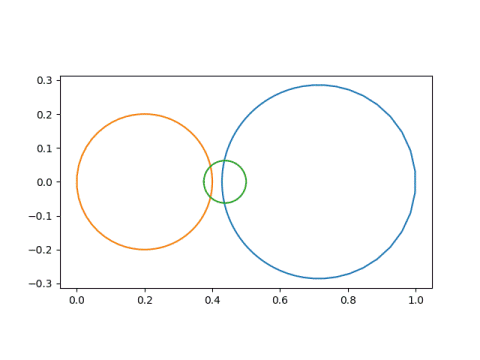Notice that the first circle, in blue, started out as the smallest circle and was contained inside the second circle, in orange. But in the image, the blue circle became the largest, and is no longer inside the orange circle. That is because our Möbius transformation has a singularity at -4/3, and things get turned inside-out around that point.

Next we’ll look at an example of lines being mapped to lines.

```    line = linspace(-100, 100, 600)
curves = [line, 1j*line - 4/3]
plot_curves(curves)
plot_curves([m(c) for c in curves])
```

This produces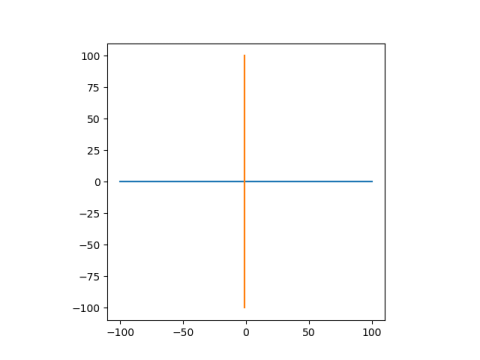and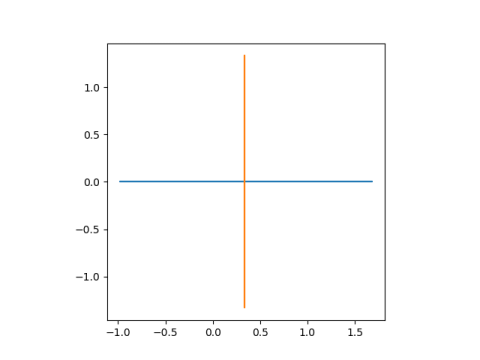These lines are mapped to lines because they both pass through the singularity at -4/3. The real axis, in blue, is mapped to itself. The line -4/3 + iy, is shifted over to have real part 1/3.

Finally, lets look at lines being mapped to circles. Since the inverse of a Möbius transformation is another Möbius transformation, this example also shows that circles can be mapped to lines.

```
lines = [1j*line - 4, 1j*line + 4, line - 4j, line + 4j]
plot_curves(lines)
plot_curves([m(c) for c in lines])
```

This produces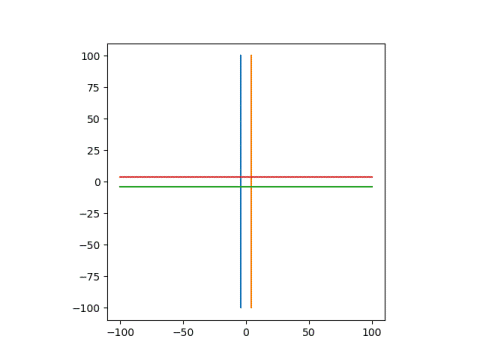and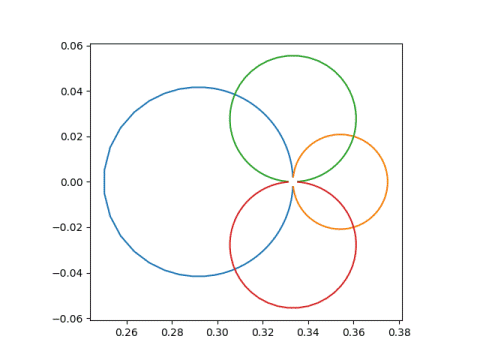Note that the circles don’t quite close. That’s because my line only runs from -100 to 100, not -∞ to ∞. The gap in the circles is at 1/3, because that’s the limit of our transformation (z + 2)/(3z + 4) as z goes to ±∞.

## Smiley faces

To illustrate things further, I’d like to look at a smiley face and what happens to it under different Möbius transformations.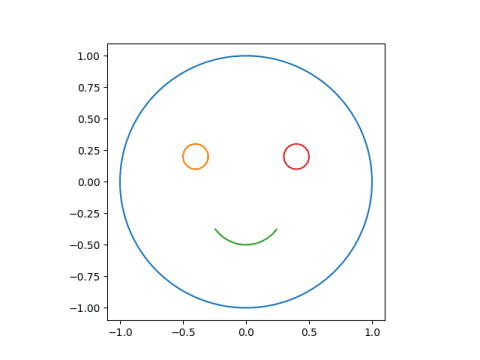Here’s the code to draw the original face.

```    dash = linspace(0.60, 0.90, 20)
smile = 0.3*exp(1j*2*pi*dash) - 0.2j
left_eye  = circle(0.1, -0.4+.2j)
right_eye = circle(0.1,  0.4+.2j)
face = [circle(1, 0), left_eye, smile, right_eye]
```

Next, let’s subject this face to the Möbius transformation with parameters (1, 0, 1, 3). The singularity is at -3, outside the face and fairly far away.Next we’ll use parameters (1, 0, 1, -1+1j), which has a sigularity at 1 – i, closer to the face, and hence more distortion.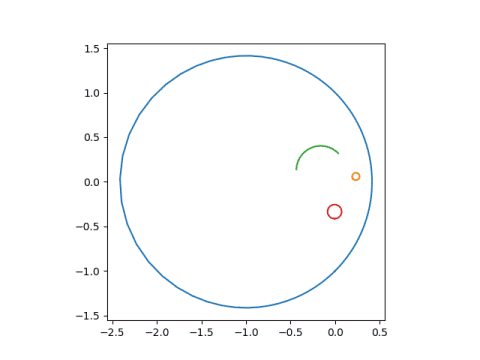Now we use parameters (1, 0, 3, 1), putting the singularity at -1/3, inside the face.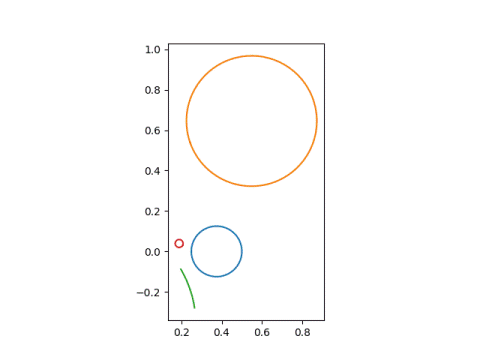Finally, we look at parameters (1, 0, 1, 0.4-0.2j), putting the singularity inside the left eye.The next post explains how to pick the parameters of a Möbius transformation to make points go where you want.

## 2 thoughts on “Circles to Circles”

1. To everyone: Have a look at the wonderful book “Indra’s Pearls: The Vision of Felix Klein” from Mumford, Series and Wright. They carry the concept to extremes (in a very beautiful and aesthetic sense).

2. Jonathan

Thanks for the recommendation, Markus.